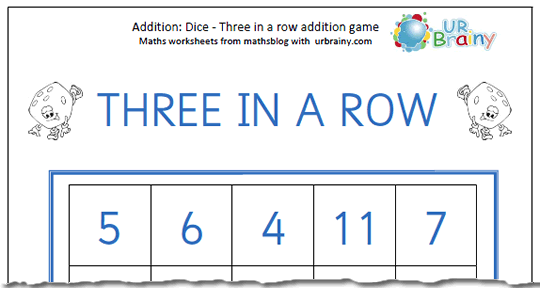## Maths for Halloween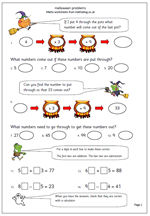More Halloween worksheets can be found on the urbrainy.com site – well worth a visit.

Nobody seems to be sure about the origins of Halloween but celebrations in the UK seem to be on the increase, perhaps following its popularity in the USA. We do know that it is always on the eve of All Saints Day and that pumpkins, apple bobbing, dressing up and demanding sweets seem to be important modern features.

So why not try a maths worksheet on Halloween? With less emphasis on the government’s planning more schools are developing themes for their maths. This page is suitable for older children who have a grasp of multiplication. A number is put through the two pots, firstly multiplying by 3 and then adding 9. The second set of questions show the resulting number and have to be worked backwards to find the number that is inputted.

Finally there are some missing digit questions. All very spooky!

Halloween

More Halloween worksheets can be found on the urbrainy.com site – well worth a visit.

## Year 6 maths worksheet: Adding fractions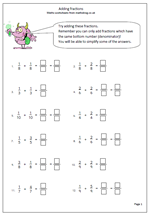One of the big stumbling blocks in maths is working with fractions, especially addition, subtraction, multiplication and division. The problem is caused by a lack of understanding of what a fraction actually means and how they can be manipulated to make the task easier. (Equivalent fractions being the key.)

A common fault when adding fractions is for a child to simply add the two top numbers and then add the two bottom numbers. This will always lead to a wrong answer and it is important to stress to children that to add two fractions the bottom numbers (denominators) must be the same. The reason for this can easily be shown by cutting a shape in to different sized parts and then asking how much two of the parts could be.

This particular worksheet takes all the stress out of the problem as the denominators are already the same for each addition question. Where an answer can be simplified a further answer is expected.

## Maths SAT Paper A 2010: Questions 17 and 18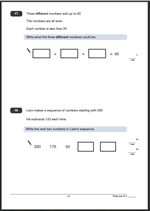Question 17 of the Maths SAT Paper A 2010 asks for three different numbers that add up to 40. Each number is less than 20 and each number is even.Surprisingly there are only four possible answers:
18 + 16 + 6
18 + 14 + 8
18 + 12 + 10
16 + 14 + 10
One mark given for any of these. The numbers can be given in any order.
Suggested method:
There are two key points here: firstly each even number is below 20 and secondly all the numbers are different.
I approached this by thinking what was the first even number below 20, which of course is 18. I took 18 from 40, leaving 22. I then thought of two even numbers that made 22. (20 and 18 had already gone) so 16 and 6 would be fine.

Question 18 is all about negative numbers and counting back in steps of 125.

Subtracting 125 from 50 is tricky and best done making notes rather than trying to write a standard subtraction method out. The best way is to subtract 50 taking the number to zero. Work out that 75 more needs to be subtracted, making -75.
Subtracting another 125 is easier now that both numbers are negative. Mentally adding 75 and 125 will give 200 so the same process with negative numbers will give -200.
Children find negative numbers quite tricky and this is a question which many will get wrong.

Questions 17 and 18 from SATs Paper A 2010

Questions 17 and 18 answers and suggested methods.

## Year 3 maths worksheet: more mental division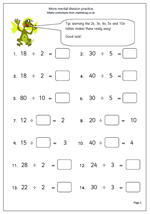This page has 14 division questions which should be answered mentally. It keeps to the easier times tables, including 2x, 3x, 4x, 5x and 10 x tables. Children who have a good knowledge of times tables should whizz through this in little time. If tables are not known it becomes a much more laborious process.

The early questions are set out in the usual form with the number to be inputted being the answer to the division calculation. The later questions have different parts of the division number sentence missing. An example:

12 divided by ? = 2

Here the relationship between division and multiplication comes into play. if it is known that 6 x 2 = 12 then this is easy.

More mental division practice

## Resource of the Week: Rounding to the nearest million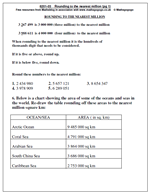One of the earliest posts that I made, back in 2007 was about rounding large numbers, up to millions. Many children are fascinated by large numbers and these two pages can help them both with reading and writing large numbers and rounding.

Thanks to mathsphere.co.uk for letting me use these resources, as they are taken from the ‘it’s All Figured Out’ CD.

Larger numbers can be rounded in just the same way as rounding hundreds or thousands; the key is to refer to the digit below the one you want to round.

Eg rounding to a million, look at the hundred thousand digit:

2 345 456 is rounded down to 2 000 000 (two million) to the nearest million because the hundred thousand digit is only 3.

2 987 654 is rounded up to 3 000 000 (three million) to the nearest million because the hundred thousand digit is 9.

There are plenty of good sources in geography, such as population figures, areas of countries etc

Rounding to the nearest million (pg 1)

Rounding to the nearest million (pg 2)

## KS2 Maths Paper 2010: Questions 16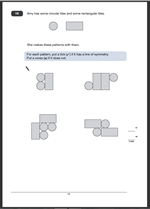Symmetry is a favourite topic for the SAT papers and this is a typical question. Four patterns of shapes are given and if a shape has symmetry mark it with a tick, if not, mark it with a cross. A maximum of 2 marks if all patterns of shapes are marked correctly. Interestingly, although it states that a tick or a cross must be used, other options will gain the marks.

Two marks are also given if:
a. If two correct are ticked and incorrect two are not marked at all.
b. If correct lines of symmetry are drawn and the other two shapes left blank.
c. If other alternative and unambiguous signs are given eg Y and N.

One mark is given if three of the four diagrams are ticked or crossed correctly.

Suggested method:
Children are usually given a small mirror to help with symmetry questions. The mirror can be placed along potential lines of symmetry and look from either side. If it is symmetrical the shape will look like the original.
Without a mirror, it is a little trickier. Probably the best way is to draw a potential line of symmetry on the shape and imagine what it would be like if folded along the line. If one half would fit exactly over the other it is symmetrical.

Question 16 from SATs Paper A 2010

Question 16 answers and suggested method

## Multiplying 2 and 3-digit numbers by 10 or 100Today I have published another multiplying worksheet, suitable for Year 4 children. Of course, multiplying by ten is easy; just move each digit one place to the left and place a zero in the units. Repeat this for multiplying by 100, but avoid the trap of saying ‘add a nought’.

This page has incomplete number sentences. Often the answer is given and the numbers to be multiplied have to be found. This leads to a little more thought and develops the relationship between multiplication and division.

This page can be found in the Year 4 Calculating section of the site.

Multiplying 2 or 3-digit numbers by 10 or 100

## Reading and writing Roman numerals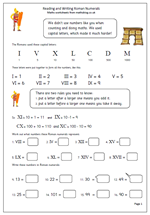Reading and writing in Roman numerals is quite tricky and if you are thinking of doing addition and subtraction with them it is much easier to convert them, do the sum and then rewrite the answer in Roman letters.
The Roman system is based round 7 letters:
I = 1
V = 5
X = 10
L = 50
C = 100
D = 500
M = 1000

Interestingly there is no zero!
Numbers can be written by writing these letters, and then adding them up. So:
XVI is 10 + 5 + 1 = 16.
There are a few rules to follow:
1. It is possible to repeat a letter many times (xxxx = 40) but a general rule is that a letter can only be repeated three times.
2. If a letter is placed after a letter of greater value then add. eg VI = 5 + 1 = 105
3. If a letter is placed before another letter of greater value subtract that amount. eg IV = 5 – 1 = 4
Roman numerals are still used in certain circumstances. You may see them on a clock face, in an index or, probably most often used for the date at the end of a film or TV programme. These two pages of worksheets explore some of the easier aspects of reading and converting Roman numerals.

These worksheets can be found in the Year 6 Understanding Number category.

## KS2 Maths Paper 2010: Questions 14 and 15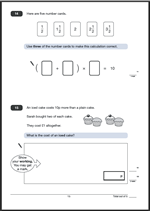Suddenly the questions become harder as questions 14 and 15 require a little more thought before trying to answer them.
With question 14 a knowledge of what happens when a number is multiplied by a half will give a clue that the most obvious answer involves using the only card without a half on (the 2) as the one outside the brackets. This means that the cards inside the bracket must add up to 5 and is just a matter of finding a pair which add up to 5.

This also assumes a knowledge that the addition inside the brackets is carried out before the multuplication.

The second correct answer given on the SATs answer booklet is much harder to work out and it would require a knowledge that ten divided by two and a half is 4 and then finding two cards that add up to 4.

Another alternative approach is to convert the fractions into decimals and this does make the multiplication easier. Interestingly a correct decimal answer is accepted for the full mark.
Question 15 is worth two marks.
If the answer is incorrect but appropriate working out has been shown, then one mark can be given.

An iced cake costs 10p more than a plain cake.

Sarah bought two of each cake.

They cost £1 altogether.

What is the cost of an iced cake?

There are several ways of looking at this. I would halve £1 to get 50p and then work out in my head two prices 10p apart that make 50p:  ie 30p and 20p.

But the SAT testers are thinking about written evidence, so another way is to say that if two of each cake cost £1 then one of each cake would cost 50p.  As the iced cake is 10p more expensive, take 10p from 50p which is 40p. Divide 40p by 2 is 20p. As the iced cake is 10p more add 10p to 20p which is 30p.
£1 ÷ 2 = 50p;         50p – 10p = 40p;        40p ÷ 2 = 20p;           20p + 10p = 30p
Another working out, using different thought processes might show:
10p x 2 = 20p;     £1 – 20p = 80p     80p ÷ 4 = 20p    20p + 10p = 30p
It must be noted here that that many children will do this more as a trial and error process and find an answer without any working out shown.

Questions 14 and 15 from SATs Paper A 2010

Questions 14 and 15 answers and suggested methods